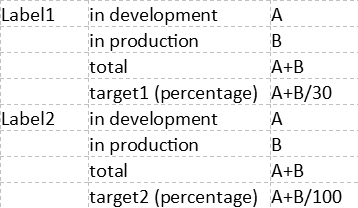# Selecting other calculation depending on sort Row

I’m hoping that there is a possibility to make a calculated field where the calculation is different, depending on different row.
I clarify:I have this calculation:
Aggregate({
[Status].,
[Status].,
[Status].})/30

for label 1 and

Aggregate({
[Status].,
[Status].,
[Status].})/100

for label 2

Is there a possibility for making 1 calculation and depending on the label the calculation does /30 or /100 (a select on the label 1 or label 2)

Hi @_P3

Yes, you can create a custom calculated measure that would behave differently depending on the member in “Label” dimension depending on the name of the displayed label.
Try this approach

``````Case WHEN
[Label].CurrentMember.name = "Label1"
THEN
Aggregate({
[Status].,
[Status].,
[Status].},
[Measures].[Issues created]
)/30
WHEN
[Label].CurrentMember.name = "Label2"
THEN
Aggregate({
[Status].,
[Status].,
[Status].},
[Measures].[Issues created]
)/100
END
``````

Martins / eazyBI support

This does the trick, thank you very much!!!